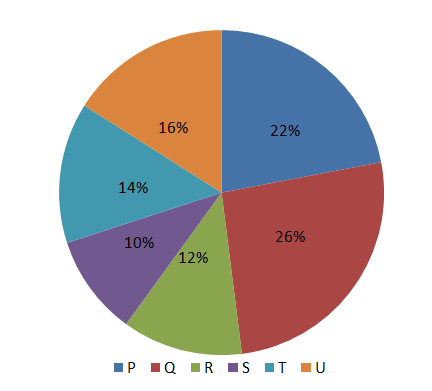Question 3

# The following pie chart provides information about the revenue share of six companies P, Q, R, S,T, U as a percentage of the total car market(in Rs.)in the year 2010. These are the only six companies producing car in the market.         Revenue shareIf the revenue share of company T increases by 20% in the year 2011, then find the percentage increase in therevenue share of these six companies in the year 2011 assuming that all the other companies except Tgenerated the same revenue as they did in the year 2010.

Solution

Let, us assume the total revenue for 2010 as 100.

Since, all the companies except T have generated the same revenue in 2011, the percentage increase in revenue for all companies will be only due to the increase in revenue of T.

Increase in revenue of T(2011) = 20% * 14 = 2.8

Total revenue(2011) = 102.8

$$\therefore$$ % increase in revenue = $$\frac{102.8-100}{100}$$ * 100% = 2.8 %

Hence, option A.